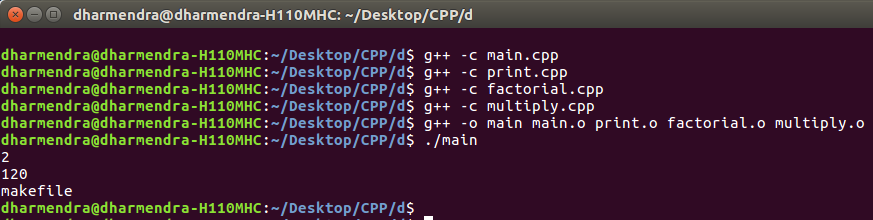# MakeFile in C++ and its applications

• Difficulty Level : Easy
• Last Updated : 02 Nov, 2018

Given a C++ program and the task is to break the entire program in the form of Makefile.
It is basically used to create .cpp file and .h file for each class/functions and its functionalities and later link them all through a Makefile command available in C++.

• It makes codes more concise and clear to read and debug.
• No need to compile entire program every time whenever you make a change to a functionality or a class. Makefile will automatically compile only those files where change has occurred.
• Generally, in long codes or projects, Makefile is widely used in order to present project in more systematic and efficient way.

Example: Create a program to find the factorial and multiplication of numbers and print it.

 `// Program to calculate factorial and ` `// multiplication of two numbers. ` `#include ` `using` `namespace` `std; ` ` `  `// Function to find factorial ` `int` `factorial(``int` `n) ` `{ ` `    ``if` `(n == 1) ` `        ``return` `1; ` `    ``// Recursive Function to find  ` `    ``// factorial ` `    ``return` `n * factorial(n - 1); ` `} ` ` `  `// Function to multiply two numbers ` `int` `multiply(``int` `a, ``int` `b) ` `{ ` `    ``return` `a * b; ` `} ` ` `  `// Function to print ` `void` `print() ` `{ ` `    ``cout << ``"makefile"` `<< endl; ` `} ` ` `  `// Driver code ` `int` `main() ` `{ ` `    ``int` `a = 1; ` `    ``int` `b = 2; ` `    ``cout << multiply(a, b) << endl; ` `    ``int` `fact = 5; ` `    ``cout << factorial(5) << endl; ` `    ``print(); ` `    ``return` `0; ` `} `

Output:

```2
120
makefile```

Use Makefile to run the above program:

• File Name: main.cpp

 `#include ` ` `  `// Note function.h which has all functions ` `// definations has been included ` `#include "function.h" ` ` `  `using` `namespace` `std; ` ` `  `// Main program ` `int` `main() ` `{ ` `    ``int` `num1 = 1; ` `    ``int` `num2 = 2; ` `    ``cout << multiply(num1, num2) << endl; ` `    ``int` `num3 = 5; ` `    ``cout << factorial(num3) << endl; ` `    ``print(); ` `} `

• File Name: print.cpp

 `#include ` ` `  `// Definition of print function is ` `// present in function.h file ` `#include "function.h" ` `using` `namespace` `std; ` ` `  `void` `print() ` `{ ` `    ``cout < ``"makefile"` `<< endl; ` `} `

• File Name: factorial.cpp

 `#include ` ` `  `// Definition of factorial function ` `// is present in function.h file ` `#include "function.h" ` `using` `namespace` `std; ` ` `  `// Recursive factorial program ` `int` `factorial(``int` `n) ` `{ ` `    ``if` `(n == 1) ` `        ``return` `1; ` `    ``return` `n * factorial(n - 1); ` `} `

• File Name: multiply.cpp

 `#include ` ` `  `// Definition of multiply function  ` `// is present in function.h file ` `#include "function.h" ` `using` `namespace` `std; ` ` `  `int` `multiply(``int` `a, ``int` `b) ` `{ ` `    ``return` `a * b; ` `} `

• File Name: functions.h

 `#ifndef FUNCTIONS_H ` `#define FUNCTIONS_H ` ` `  `void` `print(); ` `int` `factorial(``int``); ` `int` `multiply(``int``, ``int``); ` ` `  `#endif `

Commands to Compile and Run above program:

```Open Terminal and type commands:
g++ -c main.cpp
g++ -c print.cpp
g++ -c factorial.cpp
g++ -c multiply.cpp
g++ -o main main.o print.o factorial.o multiply.o
./main

Note: g++ -c filename.cpp is used to create object file.
```My Personal Notes arrow_drop_up
Recommended Articles
Page :